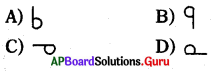Practice the AP 8th Class Physical Science Bits with Answers Chapter 10 Reflection of Light at Plane Surfaces on a regular basis so that you can attempt exams with utmost confidence.

## AP State Syllabus 8th Class Physical Science Bits 10th Lesson Reflection of Light at Plane Surfaces with Answers

Question 1.
Find the values of ∠i, ∠r in the diagram.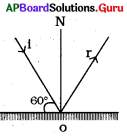A) ∠i = 60°, ∠r = 60°
B) ∠i = 60°, ∠r = 30°
C) ∠i = 30°, ∠r = 60°
D) ∠i = 30°, ∠r = 30°
D) ∠i = 30°, ∠r = 30°

Question 2.
Which of the following mirror is used as a rearview mirror?
A) Concave
B) Convex
C) Plane
D) Combination of concave and convex
B) ConvexQuestion 3.
The principle which states that light takes the path which takes the least time to travel is given by
A) Newton
B) Ampere
C) Pierre de Fermat
D) Pascal
C) Pierre de Fermat

Question 4.
In the case of a plane mirror, the object distance is ………… to image distance.
A) Equal
B) Lesser
C) Greater
D) None of these
A) Equal

Question 5.
Which of the following images cannot be caught on the screen?
A) Real
B) Virtual
C) Lateral inversion
D) None of these
B) Virtual

Question 6.
The angle of incidence is the angle between
A) The incident ray and surface of the mirror
B) The reflected ray and surface of the mirror
C) The normal to the surface and incident ray
D) The normal to the surface and reflected ray
C) The normal to the surface and incident rayQuestion 7.
The image of an object formed by a plane mirror is ………..
A) virtual
B) real
C) diminished
D) upside-down
A) virtual

Question 8.
The focal length of the plane mirror is ………….
A) zero
B) infinity
C) negative
D) positive
B) infinity

Question 9.
Two plane mirrors are kept at the following angles one by one. In which case is the number of images formed are the maximum?
A) 30°
B) 45°
C) 60°
D) 90°
A) 30°

Question 10.
When two plane mirrors are placed parallel to each other, then a number of images formed are infinite because ……….
A) Angle between plane mirrors is 0°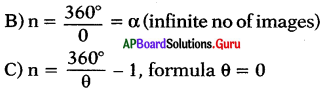D) All are correct
D) All are correctQuestion 11.
In the given figure the value of x and y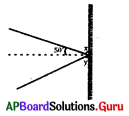A) 40°, 40°
B) 90°, 90°
C) 50°, 50°
D) 180°

Question 12.
In the given figure the value of δ is ………… degrees.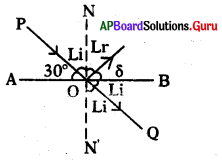A) 180°
B) 90°
C) 30°
D) 60°
C) 30°

Question 13.
The angle of reflection is equal to the angle of incidence …………
A) always
B) sometimes
C) never
D) under special conditions
A) always

Question 14.
For a plane mirror, the incorrect statement is
A) it forms a real image
B) image is as far behind the mirror as far the object is in front of it
C) the image formed is erect
D) the image formed is of the same size as the object
A) it forms a real imageQuestion 15.
Which of the following is related to plane mirrors?
i) Image is virtual and erect
ii) Object size = image size
iii) Distance from mirror to object = mirror to image
iv) Lateral inversion
A) i, ii, iii
B) ii and iii
C) i and ii
D) All are correct
D) All are correct

Question 16.
A real image
A) is formed when reflected rays do not actually meet but appear to diverge from a point
B) can’t be obtained on a screen
C) can be obtained on a screen
D) is always erect
C) can be obtained on a screen

Question 17.
Reason (R): Light always travels in a straight line.
A) A’ and ‘R’ are correct and A’ is supported by ‘R’
B) A and ‘R’ are correct, but A is not supported by ‘R’
C) A is correct, but ‘R’ is incorrect
D) ‘R’ is correct, but A is incorrect
A) A’ and ‘R’ are correct and A’ is supported by ‘R’

Question 18.
Assertion (A): Angle of incidence is equal to the angle of reflection.
Reason (R): When the light gets reflected from a surface, it selects the path that takes the least time.
A) A and ‘R’ are correct and ‘A’ is supported by ‘R’
B) A’ and ‘R’ are correct, but A’ is not supported by ‘R’
C) A is correct, but ‘R’ is incorrect
D) ‘R’ is correct, but A is incorrect
A) A and ‘R’ are correct and ‘A’ is supported by ‘R’Question 19.
Assertion (A): The size of an image seems to be increased when you move the object towards your eye from a plane mirror.
Reason (R): The angle of vision plays a role in judging the size of an object.
A) A and ‘R’ are correct and A is supported by ‘R’
B) A and ‘R’ are correct, but A is not supported by ‘R’
C) A is correct, but ‘R’ is incorrect
D) ‘R’ is correct, but A is incorrect
D) ‘R’ is correct, but A is incorrect

Question 20.
Assertion (A1): The light rays which come from our right ear get reflected from the plane mirror and reach our eye.
Assertion (A2): Our brain feels that the ray (reflected) is coming from the inside of the mirror.
These statements are related to
B) lateral inversion
C) refraction
D) Fermat’s principle
B) lateral inversion

Question 21.
Choose the correct statements regarding the laws of reflection.
i) The angle of reflection is equal to the angle of incidence.
ii) The incident ray, the normal at the point of incidence, and the reflected ray lie in the same plane.
iii) The frequency of the incident light changes.
iv) The velocity and wavelength of light change.
A) i
B) i and ii
C) ii and iii
D) iii
B) i and ii

Question 22.
Fermat principle
i) Applicable for reflection of light
ii) Light selects the path which takes the least time to travel.
iii) It is also applicable for the refraction of light.
iv) Light selects the path which takes maximum time to travel.
A) i, ii, iii
B) iii, iv, i
C) i
D) iv
A) i, ii, iiiQuestion 23.
A student is standing at a distance of 2 m in front of a plane mirror. The distance between the student and his image will be
A) 2m
B) 3m
C) 4m
D) 6m
C) 4m

Question 24.
The focal length of a concave mirror in the experiment set up shown below equals to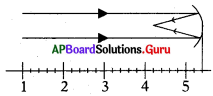A) 4 cm
B) 8 cm
C) 1.2 cm
D) 5 cm
C) 1.2 cm

Question 25.
The figure given below shows the image of a clock as seen in a plane mirror. The correct time is ………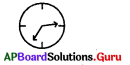A) 2:25
B) 2:35
C) 6:45
D) 9:25
D) 9:25

Question 26.
The angle of incidence between the plane of a mirror arid light ray is 45°. The angle of reflection is ……..
A) 45°
B) 30°
C) 90°
D) 60°
A) 45°Question 27.
Choose the correct mirror image of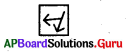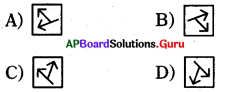A)

Question 28.
Which information do we have from the given figure?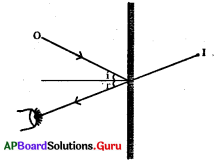i) Our brain feels that reflected ray seems to be coming from inside the mirror
ii) We can observe lateral inversion
iii) ∠i = ∠r
iv) Light travels from rarer medium falls on the smooth polished surface
A) i, ii only correct
B) i, iv only correct
C) ii, iii only correct
D) All are correct
D) All are correct

Question 29.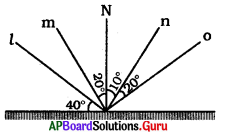Select the correct pair of incidence and reflected rays
A) (l, o) and (m, n)
B) (l, n) and (m, o)
C) (l, m) and (n, o)
D) None of the above
D) None of the above

Question 30.
If an object is placed 10 cm from the plane mirror, then the distance between the object and its image is
A) 10 cm
B) 20 cm
C) 30 cm
D) 40 cm
B) 20 cmQuestion 31.
Find the distance from O’ to I’ from the following figure if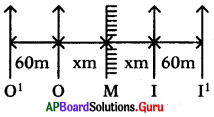A) 120
B) 240
C) 60
D) 60 + 2x
B) 240

Question 32.
What is the difference between the two images?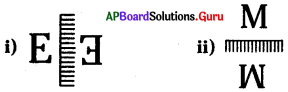A) i) is a lateral inversion
B) ii) is a vertical inversion
C) Both i) and ii) are correct
D) Can’t say